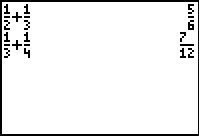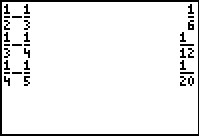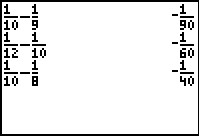# Activities

••• ##### Subject Area

• Math: Middle Grades Math: Algebraic Thinking

• ##### Author6-8

45 Minutes

• ##### Device
• TI-73 Explorer™
• TI-Navigator™

## Fraction Fun#### Activity Overview

In this activity, students will explore patterns with fractions, some of which have consecutive denominators. The TI-73 Explorer will be used to facilitate the computations so students can concentrate on patterning.

#### Key Steps

•In this activity, students will explore patterns in addition and subtraction problems with fractions. First, students look for patterns that exist when subtracting fractions with numerators of 1 and consecutive denominators.

•Next, students look for patterns in subtracting fractions with numerators of 1 and consecutive denominators.

•Finally, students explore other situations where the denominators are not consecutive numbers and the numerators may or may not be 1. In each case, students conjecture about the pattern and write the relationship algebraically.